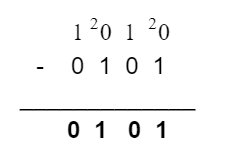# Binary Calculator

Enter the binary values and choose the required operation in the signed binary calculator to find its results.## Binary Calculator

You can perform addition, subtraction, multiplication, division, and logic functions on binary values using this calculator. Along with the results, this calculator also gives some further information about it.

## What is the binary system?

The binary system is very similar in operation to the decimal system except for the number of digits and base number.

The binary system consists of two numeric digits 1 and 0. It uses 2 as a base. The arithmetical operations are performed in the same way as the decimal system.

Some examples of the above statement are below.

## How to add binary numbers?

Adding binary numbers is similar to adding decimal numbers. Units are added into units, tens into tens, and so on. Two ones make a ten, from which zero remains in answer and one goes as a carry.

Example:

Add the binary numbers 1010 and 1111.

Solution:

1  0  1  0
+    1  1  1  1
____________
1 1  0  0  1

## How to subtract binary numbers?

Most of the method of subtracting binary numbers is similar to decimal numbers. When 1 is subtracted from 0 (i.e 0 - 1), the carry taken from the right side has the value of two. For further explanation, see the example

Example:

Subtract 0101 from 1010.

Solution: